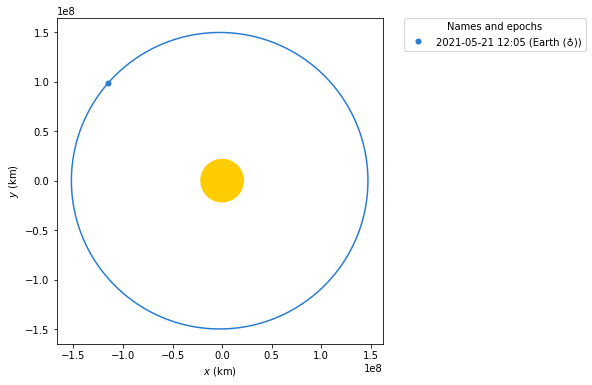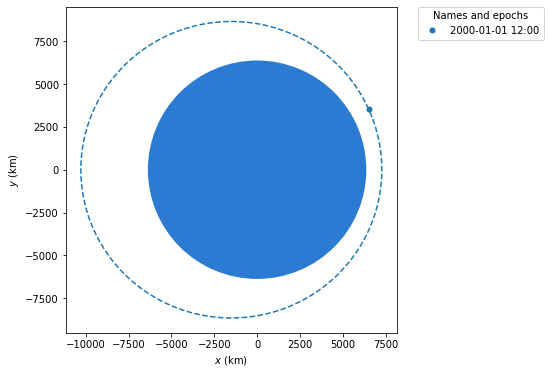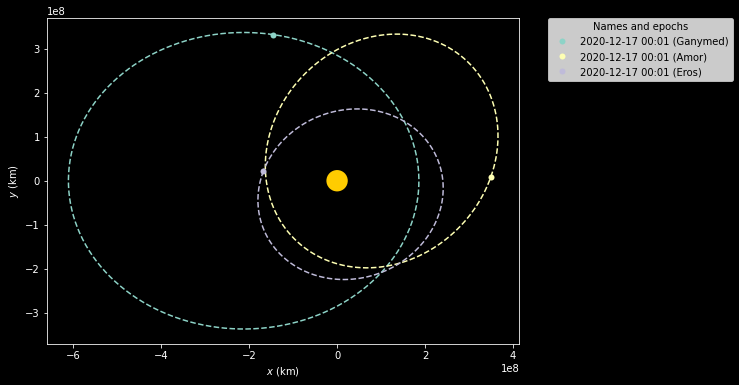# Astronomy — Ephemeris Data and Orbital Calculations¶

Several packages exist that help us work out where things are in the sky, or how to calculate their orbits, or plot trajectories between them.

We can also generate interactive 3D plots showing how different orbital planes relate to each other.

For creating educational resources, the ability to look-up reference data for planetary bodies and include it materials, as well as generating image assets associated the motion of those bodies, reduces the potential for errors.

For learners working in an interactive notebook, having access to the tools of production allows learners to create and explore their own visual assets and check their understanding or many physical problems in a visual way.

## poliastro¶

The poliastro package provides a range of tools to support interactive Astrodynamics and Orbital Mechanics activities.

For example, we can plot an orbit on a particular date:

from poliastro.bodies import Earth, Sun

from astropy.time import Time
from astropy.coordinates import solar_system_ephemeris
solar_system_ephemeris.set("jpl")

EPOCH = Time("2021-05-21 12:05:50", scale="tdb")

Earth.plot(EPOCH);Or the orbits and locations of planets in the solar system on a particular date:

from poliastro.plotting.misc import plot_solar_system

plot_solar_system(epoch=EPOCH);We can plot the orbits of various named objects:

from poliastro.examples import molniya

molniya.plot();We can also define our own orbits:

from astropy import units as u

from poliastro.twobody import Orbit

# Data from Curtis, example 4.3
r = [-6045, -3490, 2500] * u.km
v = [-3.457, 6.618, 2.533] * u.km / u.s

orb = Orbit.from_vectors(Earth, r, v)

orb

7283 x 10293 km x 153.2 deg (GCRS) orbit around Earth (♁) at epoch J2000.000 (TT)


We can then plot a 2D representation of the orbit:

orb.plot();from poliastro.plotting import StaticOrbitPlotter
from poliastro.frames import Planes

ganymed = Orbit.from_sbdb('1036') # Ganymed IAU number
amor = Orbit.from_sbdb('2001221') # Amor SPK-ID
eros = Orbit.from_sbdb('2000433') # Eros SPK-ID

#Play with the style and use a dark background
frame = StaticOrbitPlotter(plane=Planes.EARTH_ECLIPTIC, dark=True)

frame.plot(ganymed, label="Ganymed")
frame.plot(amor, label="Amor")
frame.plot(eros, label="Eros");We can also render 3D interactive orbits from a demo model:

from poliastro.examples import churi, iss

churi.plot(interactive=True, use_3d=True)